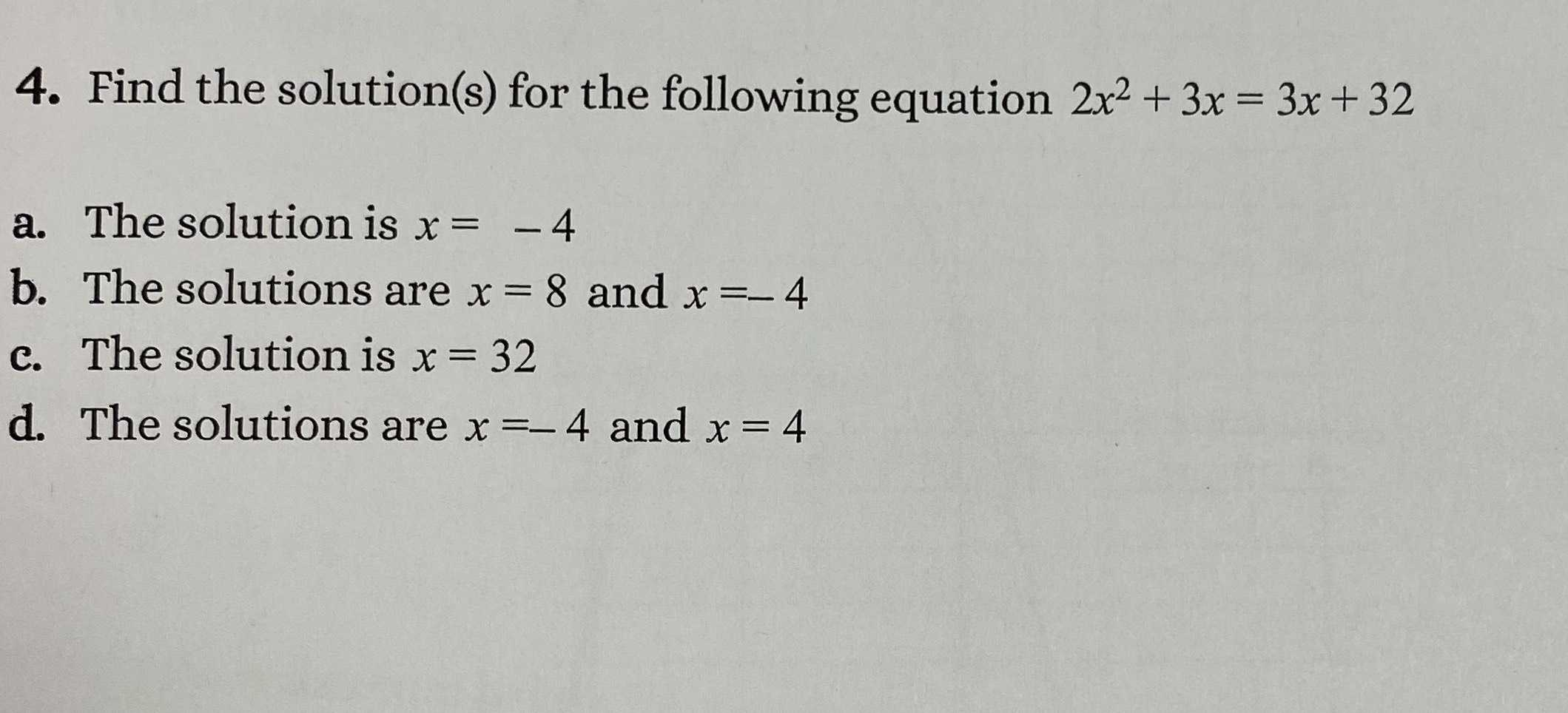### ¿Todavía tienes preguntas de matemáticas?

Pregunte a nuestros tutores expertos
Algebra
Pregunta4. Find the solution(s) for the following equation $$2 x ^ { 2 } + 3 x = 3 x + 32$$

a. The solution is $$x = - 4$$

b. The solutions are $$x = 8$$ and $$x = - 4$$

c. The solution is $$x = 32$$

d. The solutions are $$x = - 4$$ and $$x = 4$$